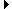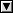Members AreaSign Up | Log In
 Free Level 2 QuotesBlogsStock AnalysisIndicator AnalysisCorrelationsMembership Benefits

 ><==>=<=()!andor+-*/Enter an indicator to analyze: characters left. Examples (close < bb[20,2,l]) and (newlow) Enter up to 5 ticker symbols to analyze: (OPTIONAL) Give your indicator a name:
 open[n]high[n]low[n]close[n]vol[n]newhigh[x,n]newlow[x,n]avevol[x,n]sma[x,n]ema[x,n]bb[x,y,z,n]faststo[k,d,r,n]rsi[x,n]imi[x,n]macd[s,f,t,r,n]smacrossover[x,y,n]smam[x,n]smamspeed[x,y,n]smamdir[x,y,n]black[n]white[n]doji[n]smbody[n]lgbody[n]smuwick[n]lguwick[n]smlwick[n]lglwick[n]gap[x,y,z,n]p1change[n]p2change[n]p3change[n]p4change[n]p5change[n]p1range[n]p2range[n]p3range[n]p4range[n]p5range[n]